American Journal of Science, Engineering and Technology
Volume 1, Issue 2, December 2016, Pages: 20-26

Remoldelling of PID Controller Based on an Artificial Intelligency (Neural Network)

Uchegbu C. E.1, Eneh I. I.2, Ekwuribe M. J.1, Ugwu C. O.2

1Department of Electrical and Electronic Engineering, Abia State Polytechnic, Aba, Nigeria

2Department of Electrical and Electronic Engineering, Enugu State University of Science and Technology, Enugu, Nigeria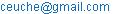(Uchegbu C. E.)

Uchegbu C. E., Eneh I. I., Ekwuribe M. J., Ugwu C. O. Remoldelling of PID Controller Based on an Artificial Intelligency (Neural Network). American Journal of Science, Engineering and Technology. Vol. 1, No. 2, 2016, pp. 20-26. doi: 10.11648/j.ajset.20160102.12

Received: September 30, 2016; Accepted: November 30, 2016; Published: December 21, 2016

Abstract: The proportional integral derivative PID controller remodeled using Neural Network and easy hard ware implementation, which will improve the control system in our industries with a high turnover. However, in this work, we propose a non-linear control of stochastic differential equation to Neural Network matching; the model has been validated, evaluated and compared with other existing controllers. The idea is to have control systems that will be able to achieve, improve, reduce waste and that is more flexible in the level of conversion, to be able to track set point change and reject load disturbance in our process industries. This paper represents a preliminary effort to design a simplified neutral network and proportional integral derivative PID control scheme, and modeling, their operational characteristics for a class of non-linear process. At the end we were able to achieve a good result by remodeling the proportional integral derivative PID controller with Neural Network Technique, and connected the plant process control where all the features of the traditional proportional integral derivative PID controller were retained and as well improved using MAT-LAB. The output was fantastic since the waste and loss encored by the process industries was drastically reduced to minimum.

Keywords: PID, Neural Network, Model, Controller, Simulation, MAT-LAB

Contents

1. Introduction

A proportional-integral-derivative controller (PID controller) is a three terms control loop controller widely used in industrial control systems.  Any time you want to control something in a process you can use a PID controller, for example: temperature, flow, pressure, speed, level, weight and so on. The controller attempts to minimize the error by adjusting the process through use of a manipulated variable. Proportional-Integral-Derivative (PID) controllers can be implemented in different ways: as a stand-alone regulator or as a distributed component of a control system. With PID controller you are given many options over the dynamics of the system, including rate response to input signal.

In many industries, neural networks have been used in many applications for control regulation, identification pattern recognition and fault detection. 

The reasons for using neural networks in industrial application controls are as follows, they can "learn" from historical patterns, i.e., they may be use as associative memories. Also they present good generalization results, i.e. it is possible to obtain appropriate outputs from patterns different to the used in the training stage. They can build input-output maps from data without known relation. They can be used with incomplete or perturbed data, because the knowledge is distributed in the interconnection weights of the network. There exist many on-line and off-line learning algorithms, which can be adapted to a particular problem.

Neural network (NN) based PID is aimed at improving computational complexity and poor real-time performance , in traditional PID control algorithm, choosing PID controller as study object, an equivalent neural network model with universal function approximating ability will be utilized to accurately remodel a known PID controller. The same plant model which was controlled by the PID controller and the equivalent neural network model will be simulated with different reference inputs, respectively.

PID controller is very poor in real-time performance by industrial computer. Therefore, to overcome its disadvantages, an equivalent NN model is designed, trained to approach a PID controller through lots of input and output data pairs form PID controller. This new method will as well improve applications PID with hardware implementation like in Digital Signal Processing (DSP).

Training an Ann

Before an ANNs can be effectively used for pattern recognition, image processing, signal processing and prediction, adaptive control and other similar problems, the network structure needs to be trained with known samples of data.  When a particular pattern is to be identified, then the ANN is first trained with the known pattern or information in form of digital signals. Then the ANN is ready to recognize a similar pattern when it is presented to the network.

The network learns or updates its weights from the given data while trying to minimize some cost function of the error between the training set data and its own output. This learning is accomplished by the back-propagation (BPN) algorithm, which is slow in convergence. It is s learning method in which an output error is reflected to the hidden layers for updating the hidden layer weights. The numerical values of the weights of the network are updated using some rule. Example of such rule is stated as below;

Once the ANN is trained, the 'learned information' is captured in these weights in a condensed and complex way. In general, these weights have no direct physical significance or meaning related to the process or phenomenon which is described or modeled by the ANN. However, the overall function of the ANN is relevant to a given task/application. A maximum of one hidden layer is often sufficient for many tasks, to attain the required accuracy. The larger the ANN, the more time it takes to train the network.

2. Design Methodology

Design a Pid controller based on neural network [NN] technique

A neural network is a powerful data modeling tool that is able to capture and represent complex input/output relationships. The motivation for the development of neural network technology stemmed from the desire to develop an artificial system that could perform "intelligent" tasks similar to those performed by the human brain. Neural networks resemble the human brain in the following two ways:

1.   A neural network acquires knowledge through learning.

2.   A neural network's knowledge is stored within inter-neuron connection strengths known as synaptic weights.

The true power and advantage of neural networks lies in their ability to represent both linear and non-linear relationships and in their ability to learn these relationships directly from the data being modeled.  Traditional linear models are simply inadequate when it comes to modeling data that contains non-linear characteristics.

The most common neural network model is the multilayer perception (MLP). This type of neural network is known as a supervised network because it requires a desired output order to learn. The goal of this type of network is to create a model that correctly maps the input to the output using historical data so that the model can then be used to produce the output when the desired output is unknown.  A graphical representation of an MLP is shown below.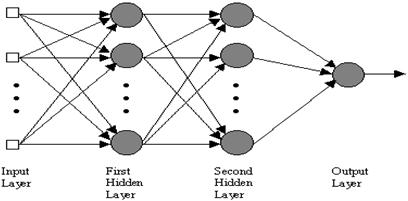Figure 1. NN Topology.

The neural network predictive controller, which is implemented in the Neural Network Toolbox software, uses a neural network model of a nonlinear plant to predict future plant performance. The controller then calculates the control input that will optimize plant performance over a specified future time horizon.

1.   The first step in model predictive control is to determine the neural network plant model (system identification).

2.   Next, the plant model is used by the controller to predict future performance. Documentation for complete coverage of the application of various model predictive control strategies to linear systems.)

Using neural network PID controller to replace the ordinary PID controllers can make the error between the system output and expected values minimum. The structure of PID control system based on neural network as shown in figure 3, while the Developed Model of PID controller based on Neural Network is shown in figure 4 respectively.

3. A Mathematicalmodel

An activation function controls the amplitude of the output. For example, an acceptable range of output is usually between 0 and 1, or it could be -1 and 1.

Mathematically, this process is described in the figure below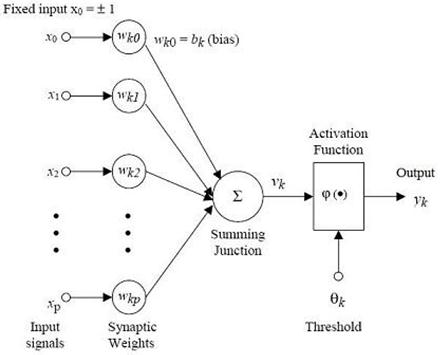Figure 2. NN Matching.

From this model the interval activity of the neuron can be shown to be: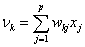(1)

The output of the neuron, yk, would therefore be the outcome of some activation function on the value of vk. 2.

General Formulation for Differential Equations

Let consider the following general differential equations which represent both ordinary and partial differential equations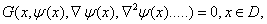(2)

Subject to some initial or boundary conditions, where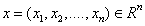denotes the domain, and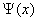is the solution to be computed.  Here, is the function which defines the structure of the differential equation and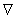is a differential operator. For the solution of the differential equation, a discredited domain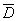over finite set of points in D is considered d . Thus, the problem transformed into the system of equations as follows:

G(x,,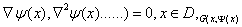.    (3)

Let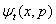denote the trail solution with adjustable parameters (weights, biases)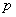, and then the problem may be formulated as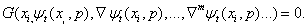(4)

Corresponding error function with respect to every input data is written as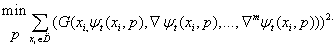(5)

Now,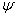may be written as the sum of two terms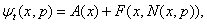(6)

Where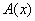satisfies initial or boundary condition and contains no adjustable parameters, whereas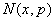is the output of feed forward neural network with the parameters and input data.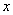The second term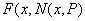makes no contribution to initial or boundary but this is used to a neural network model whose weights and biases are adjusted to minimize the error function.  .

The error computation not only involves the outputs but also the derivatives of the network output with respect to its inputs. So, it requires finding out the gradient of the network derivatives with respect to its inputs. Let us now consider a multilayered perception with one input node, a hidden layer with m nodes (fixed number of nods as proposed), and one output unit. For the given inputs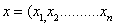, the output is given by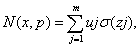(7)

Where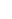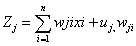denotes the weight from input unitto the hidden unit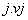denotes weight from the hidden unit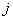to the output unit,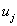denotes the biases, and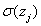is the sigmoid activation function.

The derivatives ofwith respect to input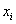is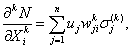(8)

Where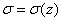and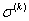denotes the kth order derivative of sigmoid function.

Let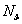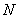denote the derivative of the network with respect to its inputs and then we have the following relation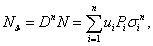(9)

Where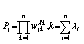(10)

The threshold is part of the controls to be adjusted; it may be incorporated as an entry of an extended synaptic matrix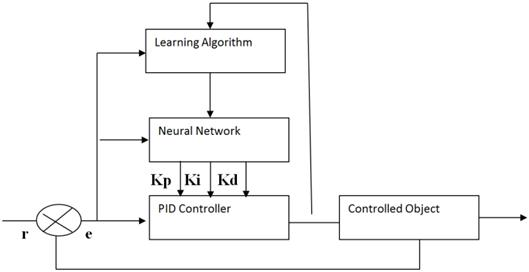Figure 3. A structure of PID Controller Based On Neural Network.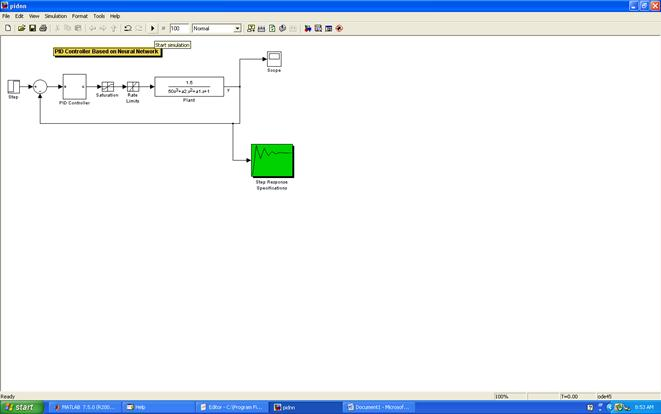Figure 4. A Developed Model of PID Controller based on Neural Network.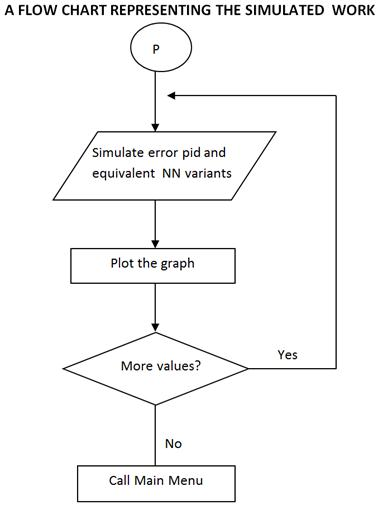Figure 5. Error PID and Equivalent NN Variants.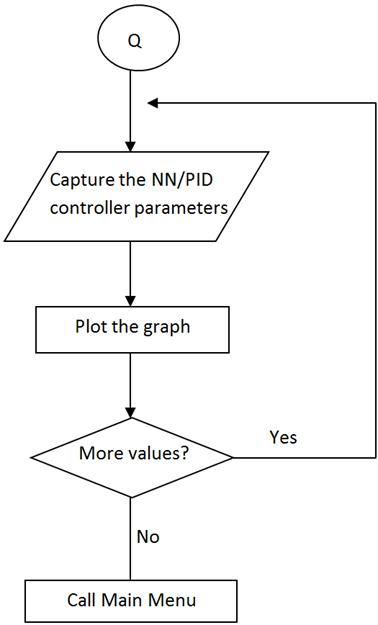Figure 6. NN/PID Control Bar Graph.

It can be seen from figure 3 and 4: above which is the structure and the model. In this case the controller consists of two parts, namely, conventional PID control and neural networks, in which, the conventional PID directly controls the controlled object with a closed loop, and its control parameters Kp, Ki and Kd. neural network is to adjust the parameters of PID controller based on the operational status of the system, to achieve a performance optimization, making the output of the output neurons corresponding to the three adjustable parameters of PID controller. Through neural network self-learning and weighting coefficient adjustment, the neural network output will corresponds to the PID controller parameters under a certain optimal control law. The flow charts in figure 5 and 6 represents the simulated work of the control system of Error PID of Equivalent NN Variants and NN/PID Control Bar Graph

5. Data Presentation and Analysis

Simulation is the imitation of the operation of a real-world process or system over time. The act of simulating something first requires that a model be developed; this model represents the key characteristics or behaviors functions of the selected physical or abstract system or process. The model represents the system itself, whereas the simulation represents the operation of the system over time. Simulation is also used when the real system cannot be engaged, and future performance is coordinated

6. Systematic Scope Graph

In order to bring the reaction to complete conversion and to prevent backward reaction, the flow rate of reactant was used as manipulated variable in the control design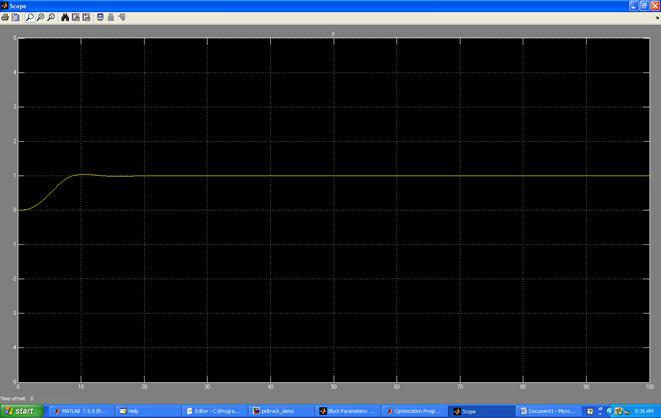Figure 7. Systematic scope graph.

Table 1. Error PID and equivalent NN variants table.

 S/N TIME OFFSET (SEC) Domain Setpoint (ms) 1 100 -1 2 200 -2 3 300 1 4 400 2 5 500 3 6 600 4 7 700 5 8 800 6 9 900 7

Table 2. NN/PID Control bar Table.

 S/N TIME NN/PID Control Bar 1 100 20 2 200 21 3 300 21 4 400 80 5 500 70 6 600 85 7 700 100 8 800 110 9 900 120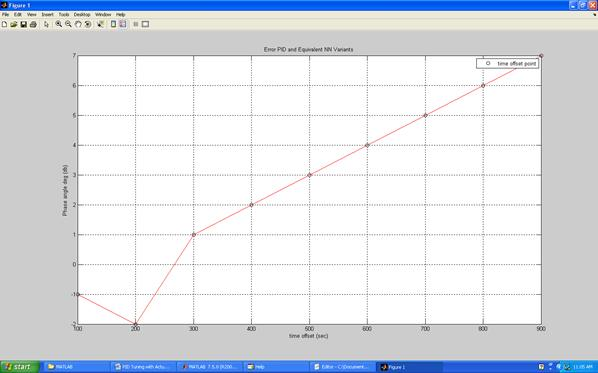Figure 8. Error PID and Equivalent NN Variants Graph.

7. Error PID and Equivalent NN in Step Input

The fig. show that error PID controller and NN equivalent which are in line for control sytem respectively, this is not without overshoot. The PID control gives serious oscillation and it did not settled throughout the simulation period.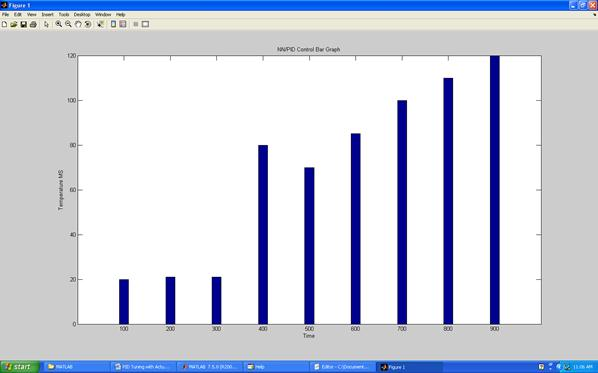Figure 9. NN/PID Control Bar Graph of Temperature Behaviour.

The fig. 9 above the first three bar chart represent a steady state of PID controlled by a neural networkwhile the subsiquent when the PID was run saperetly there a speed rise and fluctuation on the process as shown. above

8. Neural Network Modeling Result

The result views of neural network modeling in tune Time Series are grouped into two categories, tables and graphics. The details of them are described as shown above in tables 1 and 2.

9. Model Summary

Shows the summary of the neural network model and PID, from this systematic approach we can see that PID controller and neural network are paramount bar chart.

10. Conclusion

Early PID controller process takes time to compute because of it’s characteristics trait of traditional in nature, of counting the binary computing system into the continuous intervals but the problem has been overcome by the new design hardware of ANN intelligent, used to remodeled learning and recall information which make its more intelligent, very effective when used to remodeled PID controller. However remoldeling a traditional control PID makes it more flexible and it starts functioning as an intelligent system controller.

References

1. P.Atherton, Drek"Almost Six Decades in Control Engineering". Control Systems, IEEE. doi:10.1109/MCS.2014.2359588,December 2014.
2. K.NarendraandK. Parthasarathy, "Identification and Control of dynamical Systems using Neural Networks". IEEE Transactions on Neural Networks’. Vol. 1, No.1, 1990.
3. M Suzuki,T Yamamoto andT Tsuji.A design of neural-net based PID controller with evolutionary computation.IEICE Trans. Fundamentals. VOL. E87-A, No. 10, October 2004.
4. S Mall and S Chakraverty, "Regression Based Neural networktraining for the solution of ordinary differential equations,"International Journal ofMathematicalModelling and NumericalOptimisation, vol. 4, pp. 136–149,2013.
5. NSmaouiand Al-EneziS., "Modelling the dynamics of nonlinearpartial differential equations using neural networks," Journalof Computational and Applied Mathematics, vol. 170, no. 1, pp.27–58, 2004.
6. M Kumarand NYadav "Multilayer perceptrons and radialbasis function neural network methods for the solution ofdifferential equations: a survey," Computers and Mathematicswith Applications, vol. 62, no. 10, pp. 3796–3811, 2011.
7. J Mahan andK. S McFall "Artificial neural networkmethodfor solution of boundary value problems with exact satisfactionof arbitrary boundary conditions," IEEE Transactions on NeuralNetworks, vol. 20, no. 8, pp. 1221–1233,2009.
8. I.G Tsoulos, D. Gavrilis, and E.Glavas,"Solving differentialequations with constructed neural networks," Neurocomputing,vol. 72, no. 10–12, pp. 2385–2391, 2009.
9. S. A. Hodaand H. A Nagla "Neural network methods formixed boundary value problems," International Journal ofNonlinear Science, vol. 11, pp. 312–316, 2011.
10. A. J. Meade Jr. and A. A. Fernandez, "Solution of nonlinear ordinary Differential equations by feed forward neural networks," Mathematical and Computer Modelling, vol. 20, no. 9, pp. 19–44, 1994.

 Contents 1. 2. 3. 4. 5. 6. 7. 8. 9. 10.
Article ToolsAbstractPDF(2181K)